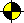Prolog Guide - Data Flow & Recursion

Guide to Prolog Programming

Home
First Steps in Prolog

### Data Flow and RecursionIn this lesson we will speak about data flow especially during manipulation with recursive data structures. Recursion is a basic technique that is used to manipulate data whose size is not known at the beginning (otherwise iteration is probably more suitable). Usually, such data are also represented using the recursive definition.

### Unary representation of numbers

Prolog has its own representation of numbers, however, for purposes of this tutorial, we define other representation of natural numbers. This simple, unary representation helps us to present a data flow during recursion. Note that it is a typical example of recursive definition where the "seed" (zero) is defined and other elements (numbers) are successively created from previously defined element.

```0   is represented as 0
N+1 is represented as s(X), where X is a representation of N```

Recursion can be naturaly expressed in Prolog (do you rember the definition of last or member in previous lesson?). So, it is easy to define a test whether a given structure is an unary number. Note again that the same procedure, i.e., unary_num, can also be used to successively generate "all" natural numbers. Try it.

```unary_num(0).
unary_num(s(X)):-unary_num(X).```

Now, we define the sum of two numbers using recursive predicate plus(X,Y,Z) (X+Y=Z).

```plus(0,X,X).                     % 0+X=X
plus(s(X),Y,Z):-plus(X,s(Y),Z).  % (X+1)+Y=Z  <==  X+(Y+1)=Z
recursion ---^      ^--- accumulator```

The plus predicate uses a data structure called accumulator to accumulate the subresult during recursive computation. Look at the following trace of computation to understand the underlying idea of accumulator.

```?-plus(s(s(s(0))),      s(0)   ,Sum).   ^ Sum=s(s(s(s(0))))
?-plus(  s(s(0)) ,    s(s(0))  ,Sum).   | Sum=s(s(s(s(0))))
?-plus(    s(0)  ,  s(s(s(0))) ,Sum).   | Sum=s(s(s(s(0))))
?-plus(      0   ,s(s(s(s(0)))),Sum).   | Sum=s(s(s(s(0))))
?-plus(      0   ,s(s(s(s(0)))),s(s(s(s(0))))). % copy accumulator to result
^-- the result is accumulated here```

It is also possible to define plus operation without accumulator using composition of substitutions.

```plus2(0,X,X).                     % 0+X=X
plus2(s(X),Y,s(Z)):-plus(X,Y,Z).  % (X+1)+Y=Z+1  <==  X+Y=Z
^--- composition of substitutions```

Look at the following trace of computation to see the difference between accumulator and composition od substitutions.

```?-plus2(s(s(s(0))),s(0),S1).          ^ S1=s(S2)=s(s(s(s(0))))
?-plus2(  s(s(0)) ,s(0),S2).          | S2=s(S3)=s(s(s(0)))
?-plus2(    s(0)  ,s(0),S3).          | S3=s(S4)=s(s(0))
?-plus2(      0   ,s(0),S4).          | S4=s(0)
?-plus2(      0   ,s(0),s(0)).  ______|
the substitutions are composed here----^```

In this particular case, the computation complexity of both approaches is similar. Also both plus and plus2 are fully declarative and they can be used to compute sum of numbers as well as to compute difference of numbers and, with some restrictions, to generate all pairs of numbers which sum is given (try ?-plus(X,Y,s(s(s(0)))).).

Following four clauses show various definitions of minus(X,Y,Z) (X-Y=Z) using plus and plus2 respectively.

```minus1a(X,Y,Z):-plus(Y,Z,X).
minus1b(X,Y,Z):-plus(Z,Y,X).
minus2a(X,Y,Z):-plus2(Y,Z,X).
minus2b(X,Y,Z):-plus2(Z,Y,X).```

Of course, minus can also be defined from scratch using recursion.

```minus(X,0,X).                      %  X-0=X
minus(s(X),s(Y),Z):-minus(X,Y,Z).  % (X+1)-(Y+1)=Z  <==  X-Y=Z```

Compare it with the next procedure minus2 which is based on another feature of minus operation. Note that minus2 requires the existence of solution (try ?-minus2(0,s(0),Z). What happens?)

```minus2(X,X,0).                     % X-X=0
minus2(X,Y,s(Z)):-minus(X,s(Y),Z). % X-Y=Z+1  <==  X-(Y+1)=Z```

### Lists (continuation)

List is another typical recursive data structure. It can be defined in a following way:

```[]    is a list
[H|T] is a list if T is a list and H is a term (member of list)```

Some examples of operations with lists can be found in previous lecture. Remind that the definition of append presented there uses composition of substitutions. In case of append it is not natural to use the accumulator.

The definiton of operation delete(X,L,DL) (deletes given element X from list L, the resulting list is DL) is also more natural to use composition of substitutions. Compare following two procedures: delete is defined using composition of substitutions while delete2 is defined using accumulator.

```delete(X,[X|T],T).
delete(X,[Y|T],[Y|NT]):-delete(X,T,NT).

delete2(X,L,DL):-del2(X,L,[],DL).

del2(X,[X|T],A,DL):-rev_append(A,T,DL).
del2(X,[Y|T],A,DL):-del2(X,T,[Y|A],DL).```
```% append a list to a reverted list, e.g. rev_append([2,1],[3,4],[1,2,3,4])
rev_append([],L,L).
rev_append([H|T],L,LT):-rev_append(T,[H|L],LT).```

In this particular case, the definition of delete is slighlty more efficient than delete2 (we omit rev_append).

The examples of append and delete does not imply that accumulator technique is not useful. The opposite is true and, in many cases, it is more natural and effective to use accumulator. The following example is the case where using accumulator is much more computationaly effective than composition of substitutions.

The procedure revert reverts given list. The definition of revert looks natural but it is not effective because of adding an element to the end of list using append in each step. revert2, which uses accumulator, is much more efficient.

```revert([],[]).
revert([H|T],Rev):-revert(T,RT),append(RT,[H],Rev)

revert2(L,RL):-rev_acc(L,[],RL).

rev_acc([],Acc,Acc).                            % reverted list is in Acc
rev_acc([H|T],Acc,Rev):-rev_acc(T,[H|Acc],Rev). % Acc contains part of list reverted until now```

Sometimes, it is possible to combine both the accumulator and the composition of substitutions to achieve the solution. Look at the definiton of halve which also exploits the build-in unification to test whether both halfs have the same length. However, testing the length in each step, which is performed by the first clause of hv, is not very effective and therefore halve is not really fast.

```halve(L,A,B):-hv(L,[],A,B).

hv(L,L,[],L).      % for lists of even length
hv(L,[_|L],[],L).  % for lists of odd length
hv([H|T],Acc,[H|L],B):-hv(T,[_|Acc],L,B).```

The following definition of halve2 is much more efficient than the original halve. halve2 also uses unification to distribute the list into two halfs but it is done once at the end of computation only. Compare both halve and halve2 on large examples (list with 100 000+ members) to see the real difference.

```halve2(L,A,B):-hv2(L,L,A,B).

hv2([],R,[],R).   % for lists of even length
hv2([_],R,[],R).  % for lists of odd length
hv2([_,_|T],[X|L],[X|L1],R):-hv2(T,L,L1,R).```

Do you know how to make the hv2 procedure even more efficient? There is one small change.

To conclude today's lecture, there are two "vacation" examples. I hope that it is clear what each of them does. Compare subpart with the definition of sublist from the previous section.

```subpart([],_).
subpart([H|T],[H|T2]):-subpart(T,T2).
subpart(L,[H|T]):-subpart(L,T).

even_odd(L,E,O):-odd(L,E,O).

odd([],[],[]).
odd([H|T],E,[H|O]):-even(T,E,O).

even([],[],[]).
even([H|T],[H|E],O):-odd(T,E,O).```

Another solution to even-odd distribution of list.

```even_odd2([],[],[]).
even_odd2([H|T],E,[H|O]):-even_odd2(T,O,E).```

Recursion is power.

 Designed and maintained by Roman Barták